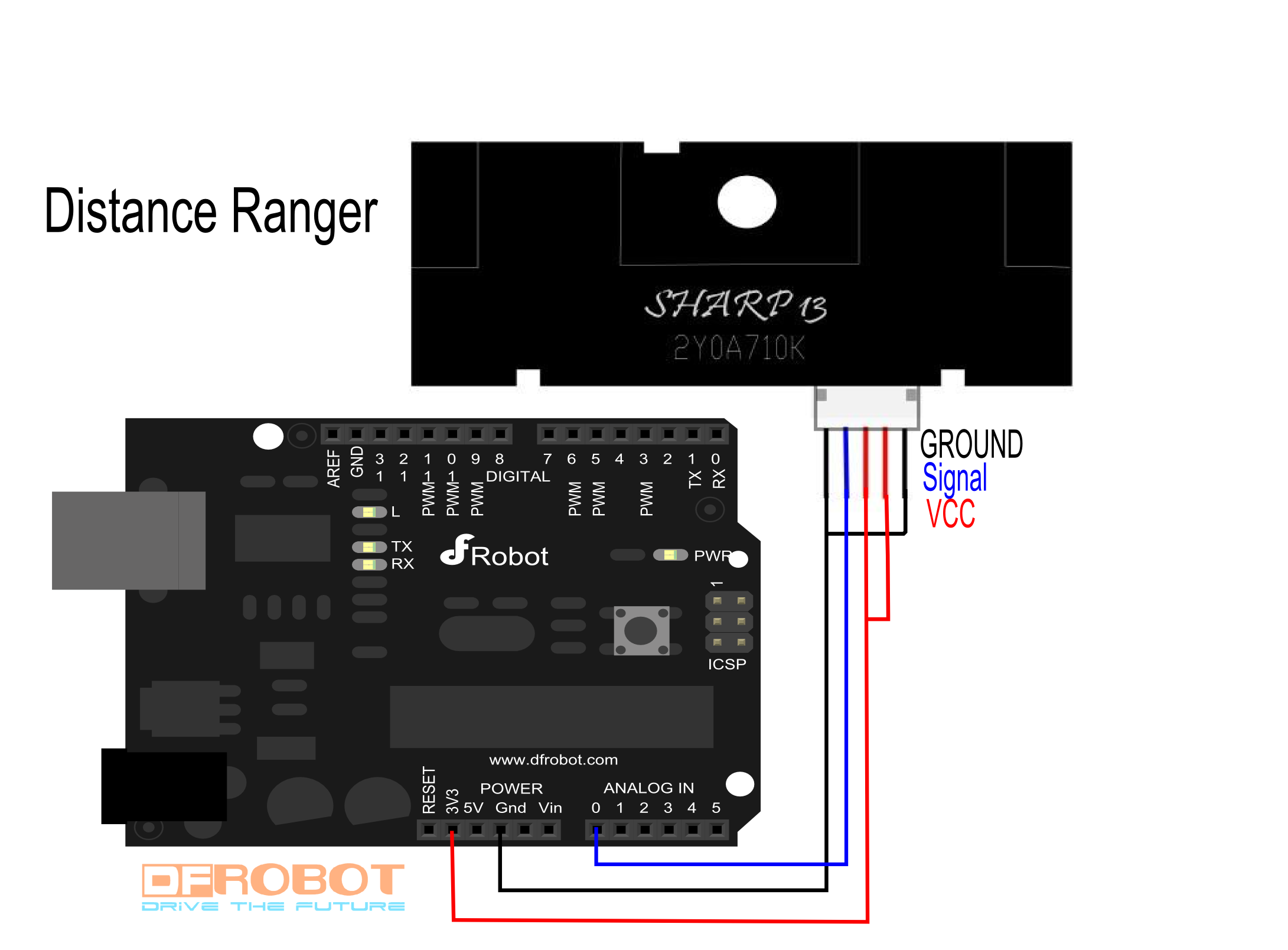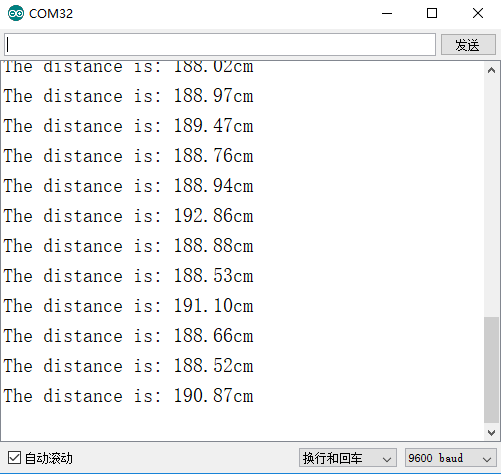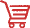Introduction

The GP2Y0A710K infrared sensor is a new version of GP2Y0A700K which takes a continuous distance reading and reports the distance as an analog voltage with a distance range of 1m (39.3") to 5.5m (216.5").

The interface is 5-wire JST connector (included with pins) with dual Vcc, dual Gnd, and a single output voltage.

Simply provide power and ground, and read a continuous analog voltage representing distance. A software lookup table can be implemented to translate the voltages to distances.

Connecting diagramUser guides

Two "voltage vs distance" diagrams are enough for using it. Voltage is linear to the inverse of distance. (Distance range is 1m to 5.5m) Two reference points:

Analog Voltage(V) Digital value(0-1023) Distance(cm)
2.5 512 100
1.4 286 500

Then, we have a linear equation of digital value and distance. (512-sensorValue)/(1/100-1/distance)=(512-286)/(0.01-0.002) => distance=28250/(sensorValue-229.5)

image:SEN0085_datasheet1.png image:SEN0085_datasheet2.png

Sample Code

/*!
* @file testDistanceSensor.ino
* @brief DFRobot's Infrared Sensor
* @n This example can print the test distance in serial port
*
*
* @author [Zhangjiawei]
* @version  V1.0
* @date  2017-6-22
* @https://github.com/DFRobot/DFRobot_IRDM_Sensor
*/

#include <Wire.h>
#include "DFRobot_IRDM_Sensor.h"

DFRobot_IRDM_Sensor DIS(A0);      //change the parameter selection pin

void setup() {
Serial.begin(9600);
}

void loop() {
float Distance;
Distance = DIS.getDistance();  //  get Distance
Serial.print("The distance is: ");
Serial.print(Distance);
Serial.println("cm");
delay(500);
}

Expected ResultsFAQ

For any questions, advice or cool ideas to share, please visit the DFRobot Forum.

More DocumentsGet Sharp GP2Y0A710K Distance Sensor (100-550cm) from DFRobot Store or DFRobot Distributor.